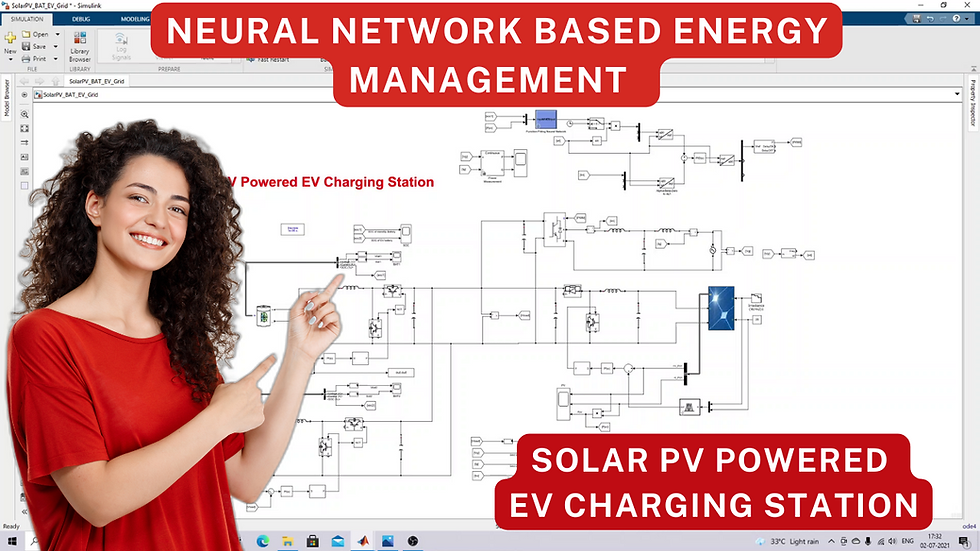top of page
Search
•Vishnupriya murugesan

# Solar PV powered EV charging station in MATLABSolar PV powered EV charging station in MATLAB

Hi viewers welcome to LMS solution!

Today we are going to see about matlab simulation of solar PV powered EV charging station thanks for watching our videos kindly subscribe our channel and also click bell icon for up notification about upcoming videos so this is the block diagram for a solar powered ev charging station so this block diagram consists of pv array along with the dc-dc converter and the mppt algorithm and then standby storage element nothing but battery storage stationary storage element along with the bi-directional dc-dc converter with dc bus voltage controller and they have grid integration grid supply and then it has ev battery with the dc-dc converter okay so in this block diagram means what's working of the ev charging station right so whatever power available in the pv this goes to charge the stationary storage element and also whenever the ev battery ev connected to the charging station right then it going to supply the power to the ev battery as well as going to charge the stationary battery and if suppose there is no power in the solar pv, there is no power generations in pv panel okay during that time on the stationary battery going to be supply the power to the charge ev battery and if suppose there is no power in the pv and then the SOC of the stationary batteries is very low then we can get the power from the grid to charging this stationary battery as well as charge the ev battery and also if suppose we have excess power in the solar pv system and then the battery soc of this battery that mean we have enough charge in the battery then during that time this is going to be this both the PV and this stationary battery going to supply the grid as well as charge the ev batteries okay so this is working on this solar powered ev charging station concept that mean so by using the solar pv array we are going to charge the battery so this is ultimate aim of this work okay today we are going to see about the matlab implementation of this solar pv powered ev charge station so let's let's start with that simulation so this is the simulation we created for a solar pv powered ev charging station right so it consists of solar pv array along with the boost converter right so this is solar pv array and then this is boost converter okay and then we are using ANFIS MPPT here this is ANFIS MPPT it's received two inputs one is irradiation another one is temperature so based upon this irradiation temperature it provide the voltage at maximum power point okay so here we are measuring the pv voltage so this pv voltage is compared with this voltage from that mean voltage reference from the ANFIS MPPT and then it's going to process via PI controller and then process via PWM generator to generate the pulse for this igbt to extract the maximum power for the pv panel okay so here we are another block scope block right so this is used for measuring the pv voltage current and power okay so this is the arrangement of the solar pv system in the solar powered ev charging station.